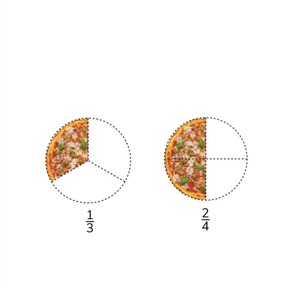# Adding fractions with different denominators

I can add fractions with different denominators.8,000 schools use Gynzy92,000 teachers use Gynzy1,600,000 students use Gynzy

## General

In this lesson, students will learn how to conceptualize and add fractions with different denominators.

## Common core standard

CCSS.Math.Content.5.NF.A.1

## Learning Objective

Students will be able to add fractions with different denominators.

## Introduction

As a class, work to see if students can find the lowest common denominator for the fractions given.

## Instruction

Show students two pizzas, each representing a different fraction. Discuss the steps required to add the two unlike fractions. First, you must create a common denominator, and then you will be able to add the two fractions. Show students how to create a common denominator for the two fractions and how to add the fractions together. Practice solving a few problems as a class. Next, show students what to do if the fractions are mixed fractions involving a whole number. Tell students to ignore the whole number until the fractions have been given a common denominator, and then to add. Remind students that if their fraction creates a mixed number that the whole number is added to the whole numbers in the problem. Practice adding fractions with different denominators. Ask students to explain their thinking. Give students fractions in a story problem. Ask them which numbers they need to solve the given problem and ask them how to solve the story problem.

## Quiz

Students will determine if a given fraction has been simplified correctly and if given fractions can be added together. They will also determine which fractions can be added together and add the fractions.

## Closing

Remind students that you cannot add fractions that have different denominators, but that you must first find a common denominator to add the fractions. Close with a few more exercises to check for understanding and be sure to ask students to explain their thinking.

### The online teaching platform for interactive whiteboards and displays in schools

• Save time building lessons

• Manage the classroom more efficiently

• Increase student engagement